# C语言博客作业04--数组

| 这个作业属于哪个班级 | C语言--网络2012 |
| ---- | ---- | ---- |
| 这个作业的地址 | C博客作业04--数组 |
| 这个作业的目标 | 学习数组相关内容 |
| 姓名 | 李兴果 |

# 0.展示PTA总分：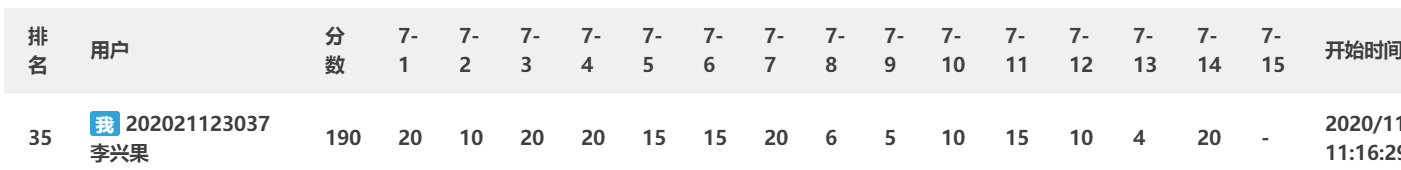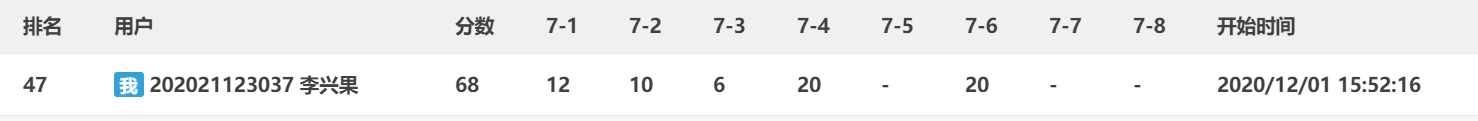# 1.本章学习总结

## 1.1 学习内容总结

• 一维数组
数据类型 数组名称[长度];
1、 数据类型 数组名称[长度n] = {元素1,元素2…元素n};

2、 数据类型 数组名称[] = {元素1,元素2…元素n};

3、 数据类型 数组名称[长度n]; 数组名称 = 元素1; 数组名称 = 元素2; 数组名称[n-1] = 元素n;

• 注意：

1、数组的下标均以0开始；

2、数组在初始化的时候，数组内元素的个数不能大于声明的数组长度；

3、如果采用第一种初始化方式，元素个数小于数组的长度时，多余的数组元素初始化为0；

4、在声明数组后没有进行初始化的时候，静态（static）和外部的数组元素初始化元素为0；

• 字符串数组
5.所有变量所占的字节大小必须相等

1、char 字符串名称[长度] = "字符串值";

2、char 字符串名称[长度] = {'字符1','字符2',...'字符n','\0'};

• 注意：

1、[]中的长度是可以省略不写的；

2、采用第2种方式的时候最后一个元素必须是'\0'，'\0'表示字符串的结束标志；

3、采用第2种方式的时候在数组中不能写中文。

• 字符串数组注意
1、strlen()获取字符串的长度，在字符串长度中是不包括‘\0’而且汉字和字母的长度是不一样的
2、strcmp()在比较的时候会把字符串先转换成ASCII码再进行比较,返回的结果为0表示s1和s2的ASCII码相等,返回结果为1表示s1比s2的ASCII码大,返回结果为-1表示s1比s2的ASCII码小
3、strcpy()拷贝之后会覆盖原来字符串且不能对字符串常量进行拷贝
4、strcat在使用时s1与s2指的内存空间不能重叠，且s1要有足够的空间来容纳要复制的字符串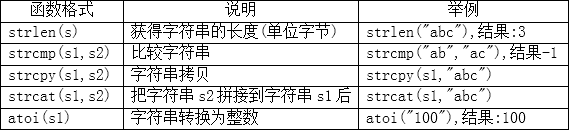• 多维数组
数据类型 数组名称[常量表达式1][常量表达式2]...

• 注意：
（1）二维数组定义的时候，可以不指定行的数量，但是必须指定列的数量
（2）多维数组的每一维下标均不能越界

## 1.数组中如何查找数据

### （1）顺序查找

1.从表中的第一个元素开始，依次与关键字比较。
2.若某个元素匹配关键字，则查找成功。
3.若查找到最后一个元素还未匹配关键字，则 查找失败。

### （2）二分查找（折半查找）

• 列如：

low=0；high=10-1；mid = (low+high)/2；

3 5 7 8 22 33 47 55 56 60
0 1 2 3 4 5 6 7 8 9

a = 22,比当前key值小，所以我们在右半边查找，令low = mid + 1，high不变；

a = 55,比当前key值小，所以我们在右半边查找，令low = mid + 1，high不变；

## 2.数组中如何插入数据

### 插入数据

for(i=0;i<10;i++)
{
if(a[i]>x)
break;
}
for(j=9;j>=i;j--)
{
a[j+1]=a[i];
}
a[i]=x;



for(i=9;i>=0;i--)
{
if(a[i]>x)
a[i+1]=a[i];
else
a[i+1]=x;
break;
}


## 3.数组中如何删除数据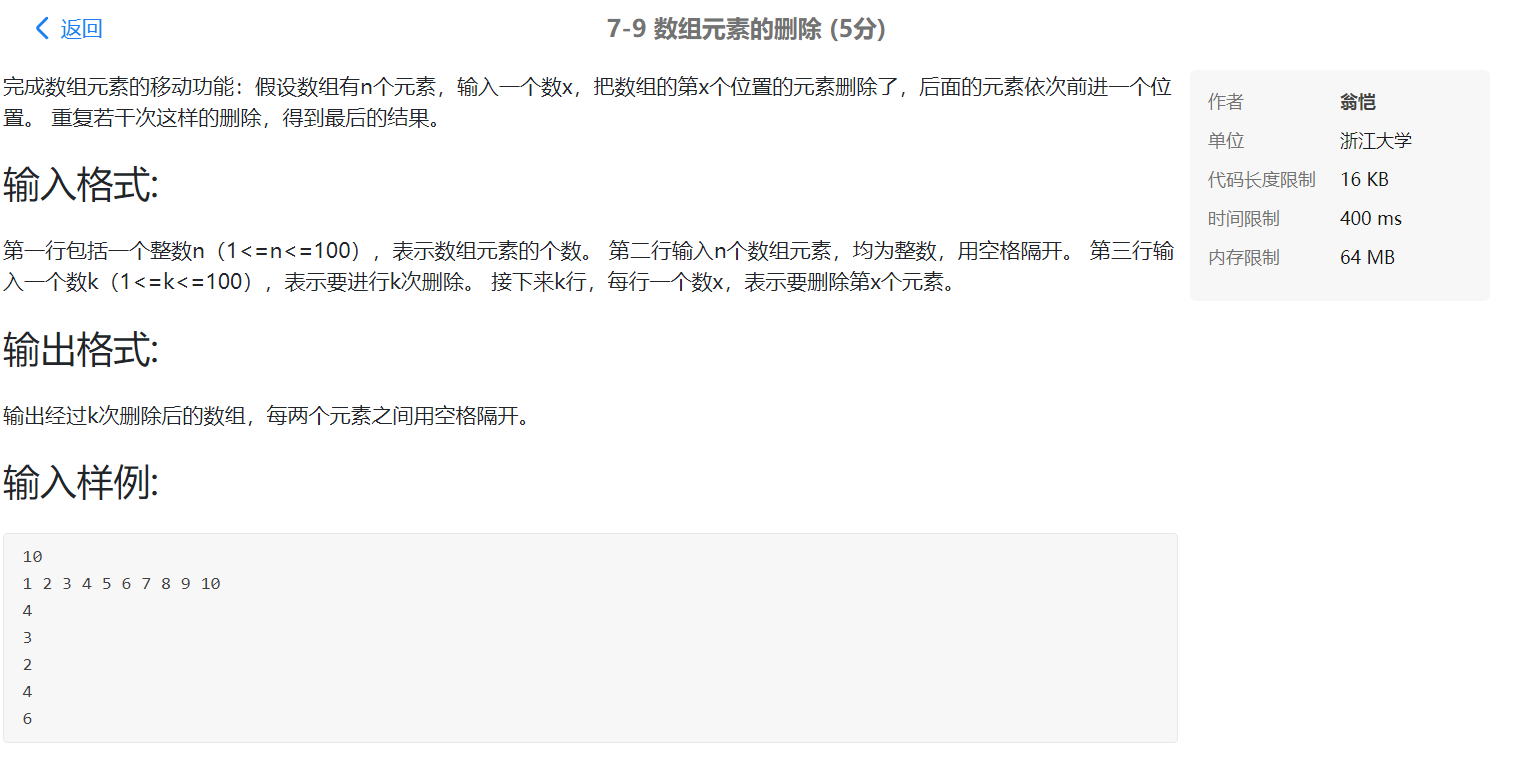定义数组a

for i=0 to i<n i自增
输入数组a[i]
输入换行符'\n'
输入删除次数k
for i = 0 to i < k i 自增
输入要删除数的位置m
for j = m - 1 to j < n j自增 //j的赋值应该为m-1，实际删除的是数组的前一个数
a[j]=a[j+1] //将后面的数往前移动
for i = 0 to i < n - k i自增
if (不是flag)
输出数组前面无空格a[i]
flag自增//记录删除次数
否则
输出前面有空格a[i]
end for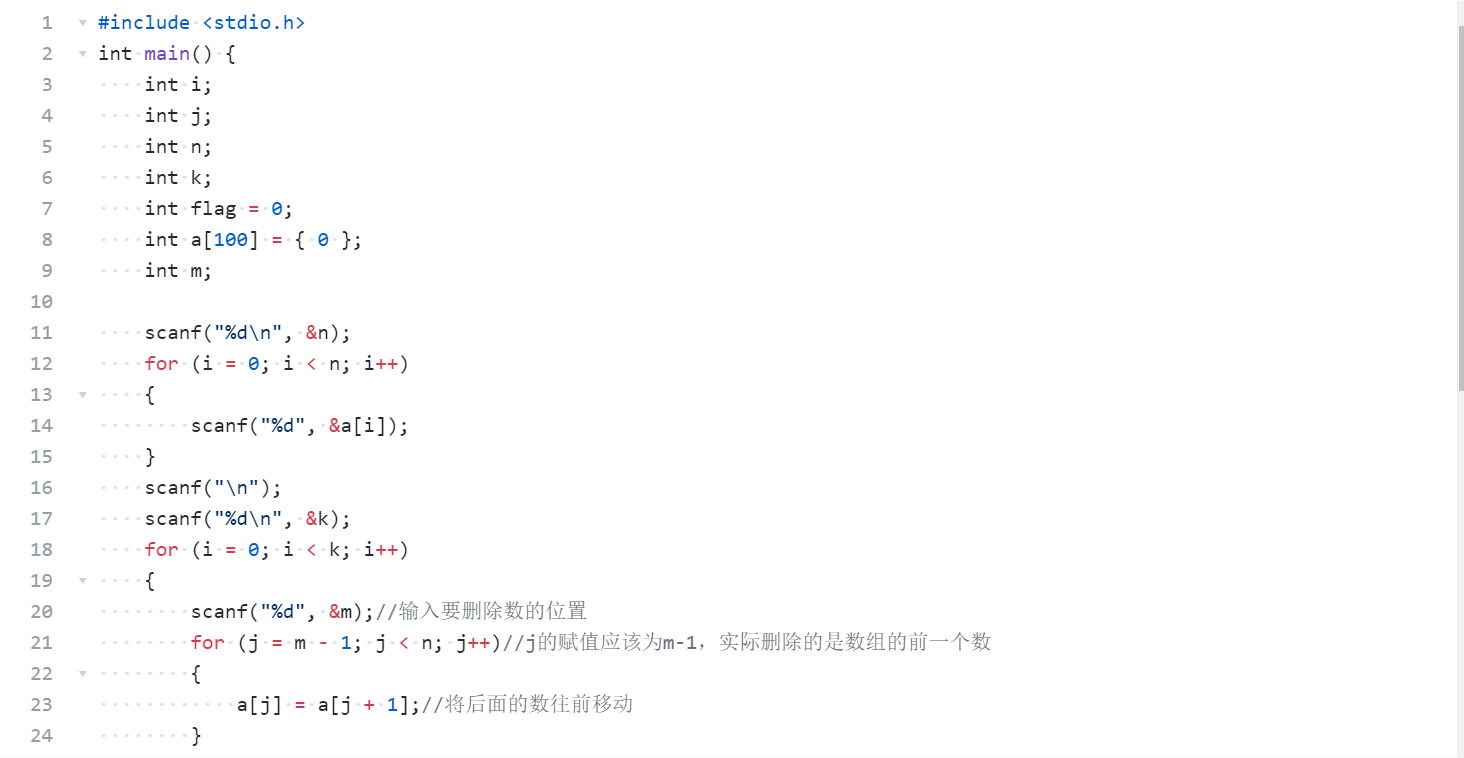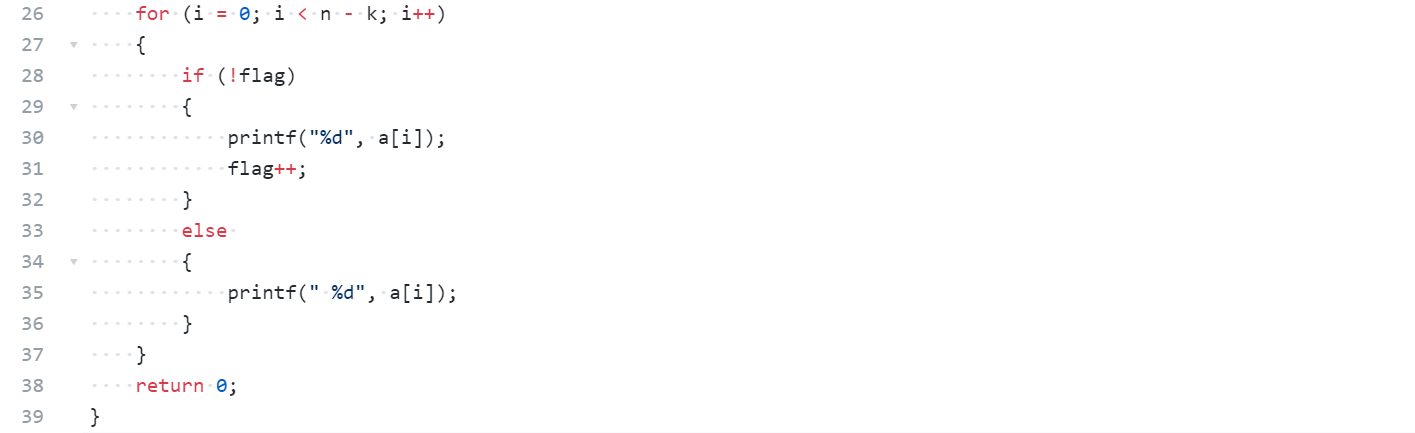## 4.数组中目前学到排序方法，主要思路？

### 1.冒泡排序: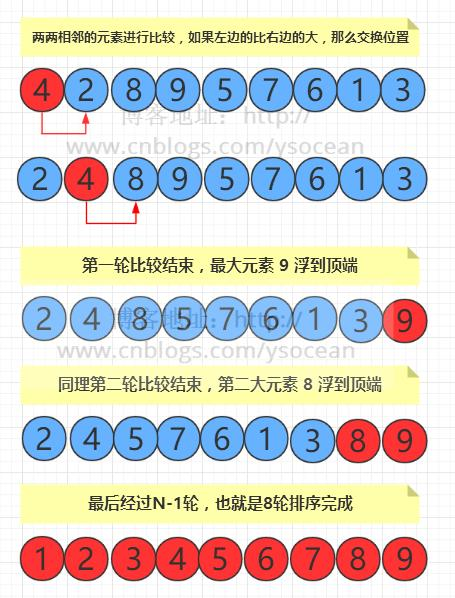for（int i=0;i<n-1;i++)
for(int j=0;j<n-1-i;j++)
if(a[j]>a[j+1])
{
int temp=a[j];
a[j]=a[j+1];
a[j+1]=temp;
}


### （1）选择排序: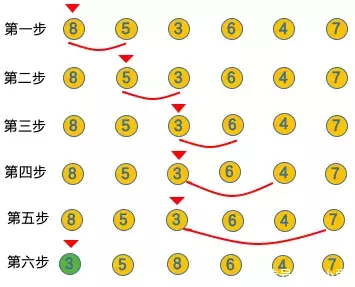for(int i = 1;i < n;i++)
{
for(int j = 0;j < n - 1;j++)
{
if(a[j] < a[j + 1])
{
temp= a[j];
a[j] = a[j + 1];
a[j + 1] = temp;
}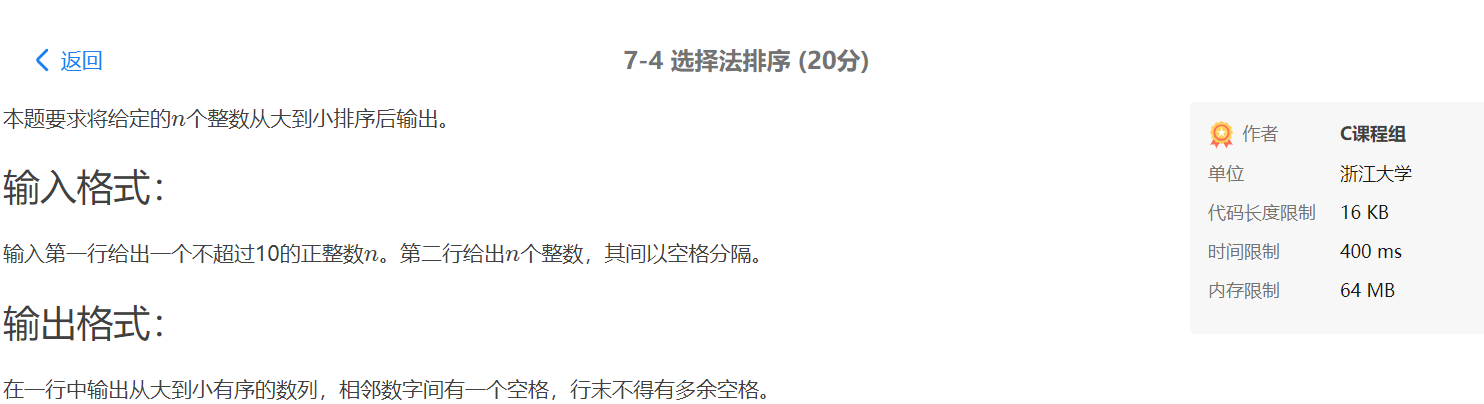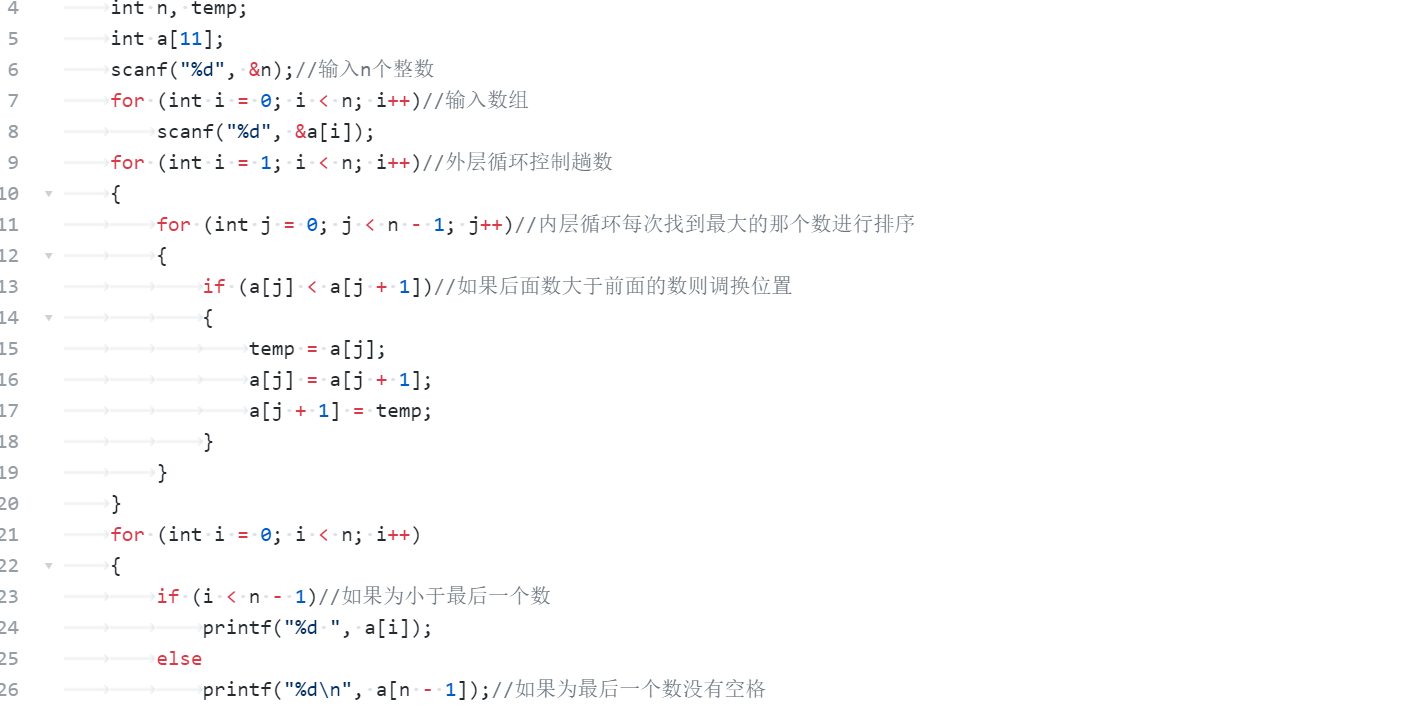### （2）插入排序：

while (j >= 0 && a[j - 1] > x) {//一般插入排序是j>1开始，这里就插一个元素，可能插0，要从0开始
a[j] = a[j - 1];//把j-1位置的元素移到j位置
j--;
}
a[j] = x;


## 6.哈希数组用法

1.有重复的数据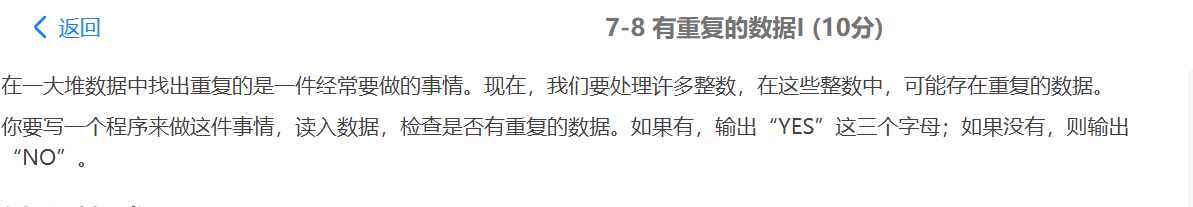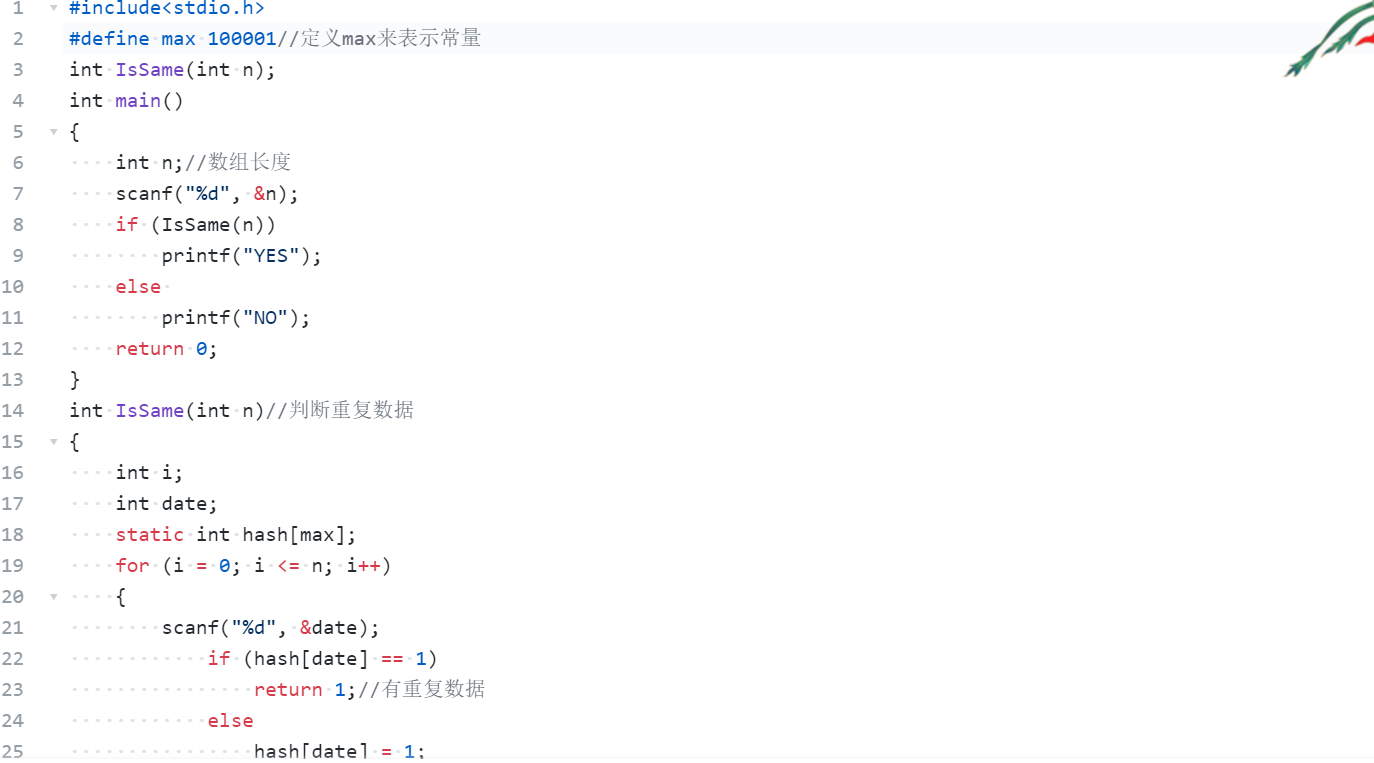## 7.字符数组、字符串特点及编程注意事项。

（1）scanf函数无法接受无字符
（2) 如果字符串的长度大于 10，那么就存在语法错误。这里需要注意的是，这里指的“字符串的长度”包括最后的 '\0'。也就是说，虽然系统会自动在字符串的结尾加 '\0'，但它不会自动为 '\0' 开辟内存空间。所以在定义数组长度的时候一定要考虑 '\0'。
（3) 如果字符串的长度小于数组的长度，则只将字符串中的字符赋给数组中前面的元素，剩下的内存空间系统会自动用 \0' 填充。

# 2.PTA实验作业

## 2.1 题目名1

2.1.1 伪代码

/*冒泡法解题思路：将相邻两个数比较，将小的数调到前头*/
/*两个循环。外层循环限制有多少趟的比较，内层循环限制某趟比较要进行两两对比的次数*/
/*如果a[i]>a[i+1],则要将两数进行交换，要求从小到大排列*/
//最多执行n-1趟，第j次比较中要进行n-i次两两比较,最大的数已经沉底

输入n，k//输入两数n几个数，k扫描遍数
for  i = 0 to i < n； i++//输入数组
scanf("%d", &a[i]);

for i = 0 to i < k; i++//外层循环限制趟数比较
{
for (j = 0; j to n-i-1; j++)//内层循环就是实行n-i次两两比较,n-1到最后一个数
{
if (a[j] 大于 a[j + 1])//实现两数的比较，如果前面大于后面的数就把它调到后面去
{
temp = a[j];
a[j] = a[j + 1];
a[j + 1] = temp;
}
}
}
for (i = 0 to i < n; i++)//实现输出
{
if (i==0)//当数据为第一个数，数组下标为0无空格
输出前面无空格数组a[i];
else
输出’空格‘ a[i];
end for



2.1.2 代码截图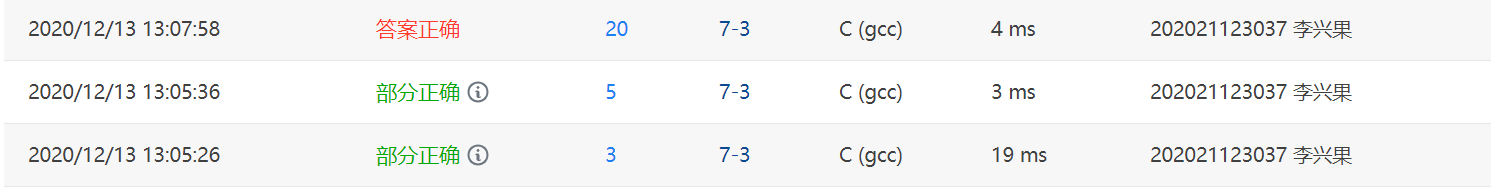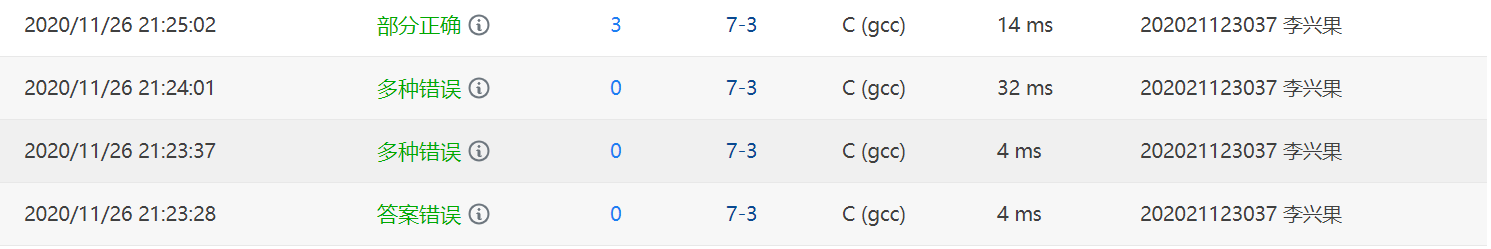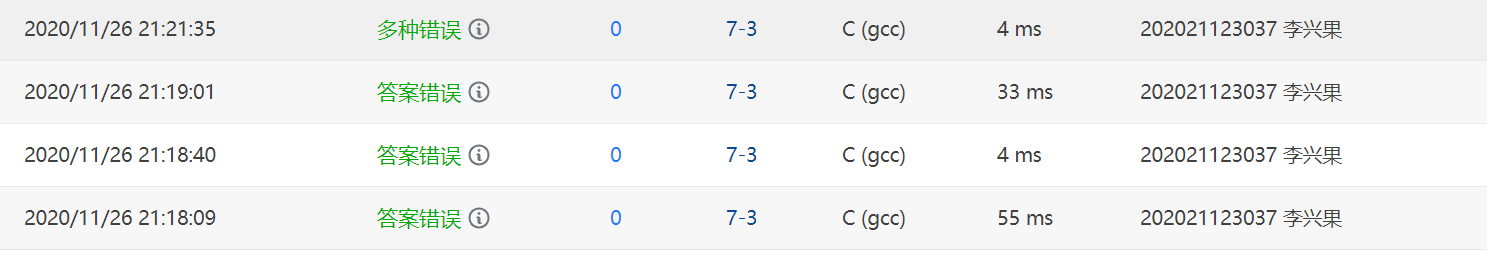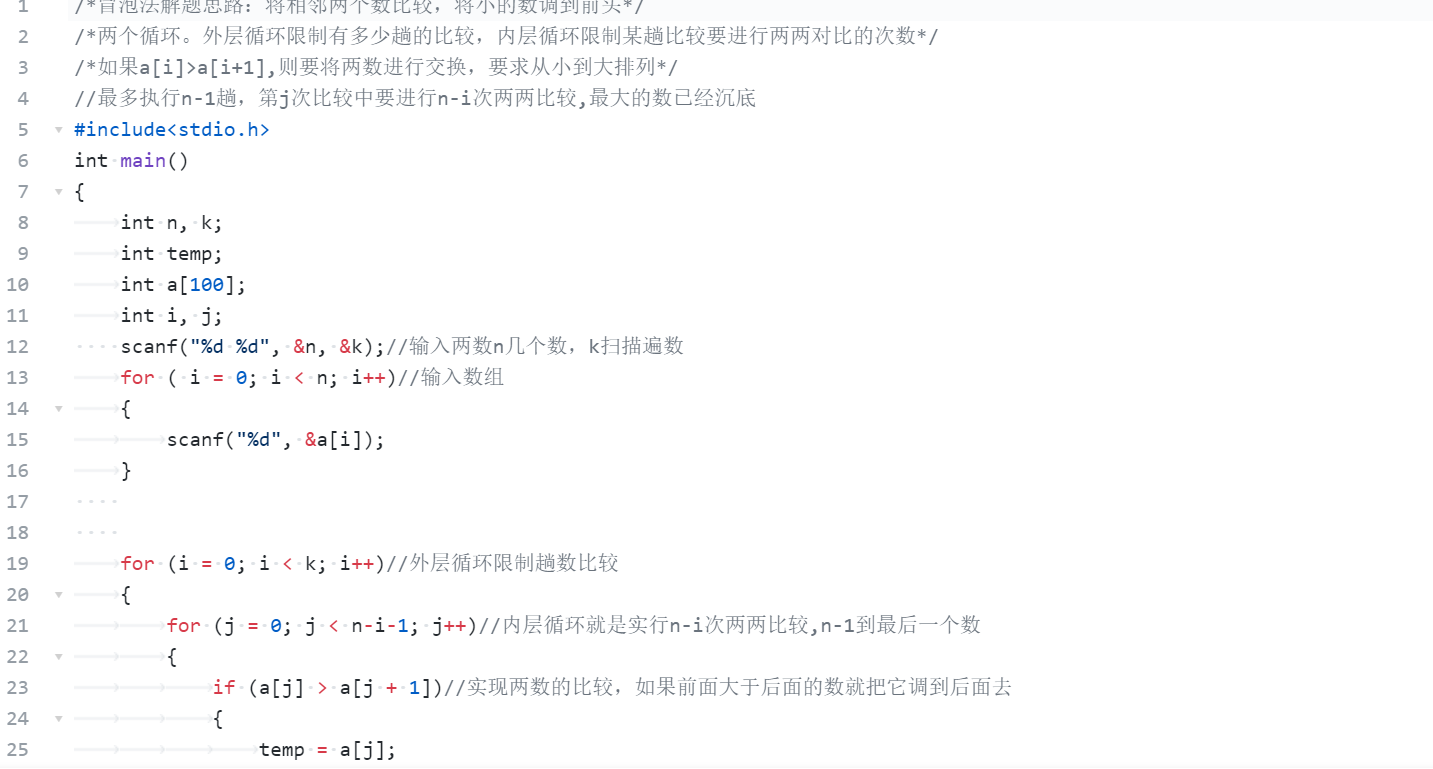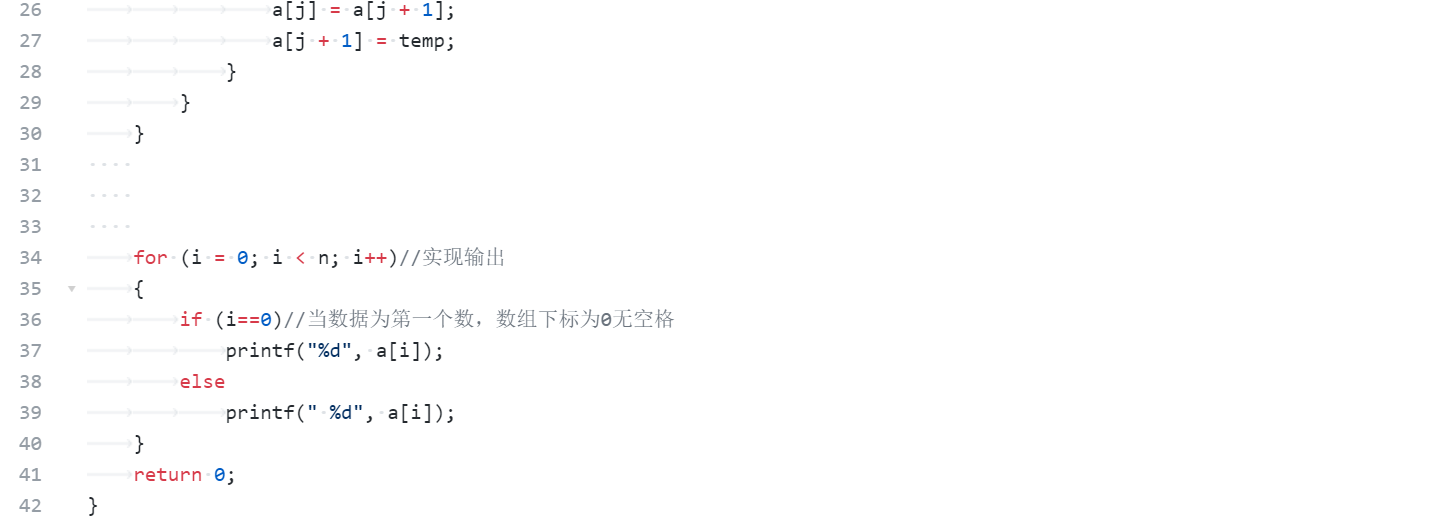2.1.3 找一份同学代码（尽量找思路和自己差距较大同学代码）比较，说明各自代码特点。

## 2.2 题目名2

2.2.1 伪代码

定义变量 b, c, d;  //储存下标：b和d储存最大值的下标，c储存最小值的下标

//第一层循环遍历每一行
for i = 0 to i < m do
先该行中的第一个数赋值给最大值并保存其位置
b = i;//保存每行第一例的位置
d = 0;//第一列
for j = 0 to j < n do//找出每一行中的最大值的值及位置
//将最大值赋值给max
b = i;//保存它的行位置
d = j;//保存它的列位置
//将找到的最大值赋值给最小值，作为初值并存储下标（因为列是一样的所以只需要储存行的位置）
c = b;//储存最小值下标
//从找到的最大值中的那一列找该列的最小值并存储其位置
for k = 0 to k < m do //从找到的最大值中的那一列找该列的最小值并存储其位置
c = k;
//判断最大值和最小值的位置是否相同
if (b == c)
flag = 1;
跳出循环、
end for


2.2.2 代码截图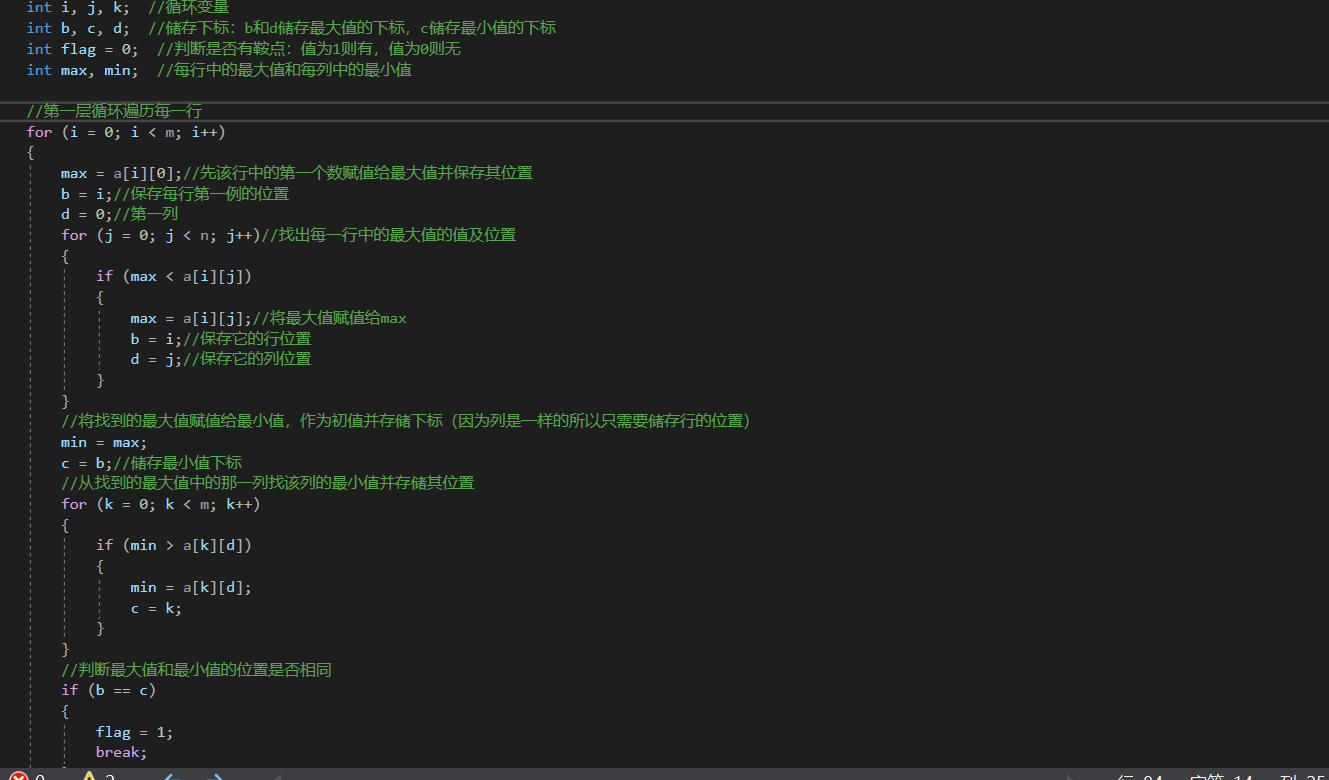2.2.3 请说明和超星视频做法区别，各自优缺点。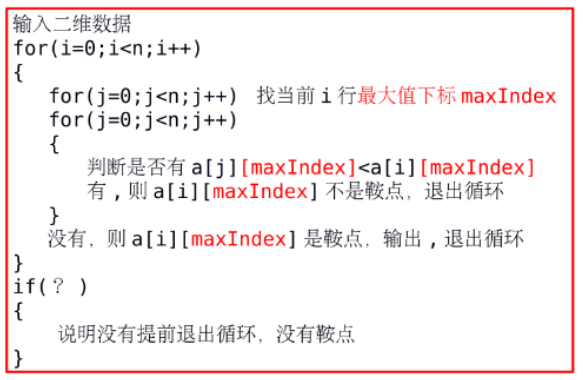• 使用break，提前退出循环，使程序更加有效率

## 2.3 题目名3

2.3.1 伪代码

定义flag

for (i = 0 to str[i] != '\n' do
（第一处为第一个字符是正负号时）
end if
if（如果是数字或者小数点的话判断下一位是否是小数点或者数字）
end if
else if（负数的判断 前一位是数字还是其他符号）
else if（正数的判断 数字前一位是不是‘（’）
end for


2.3.2 代码截图
（寻求了同学的帮助，此代码为同学的代码），自己是看同学的理解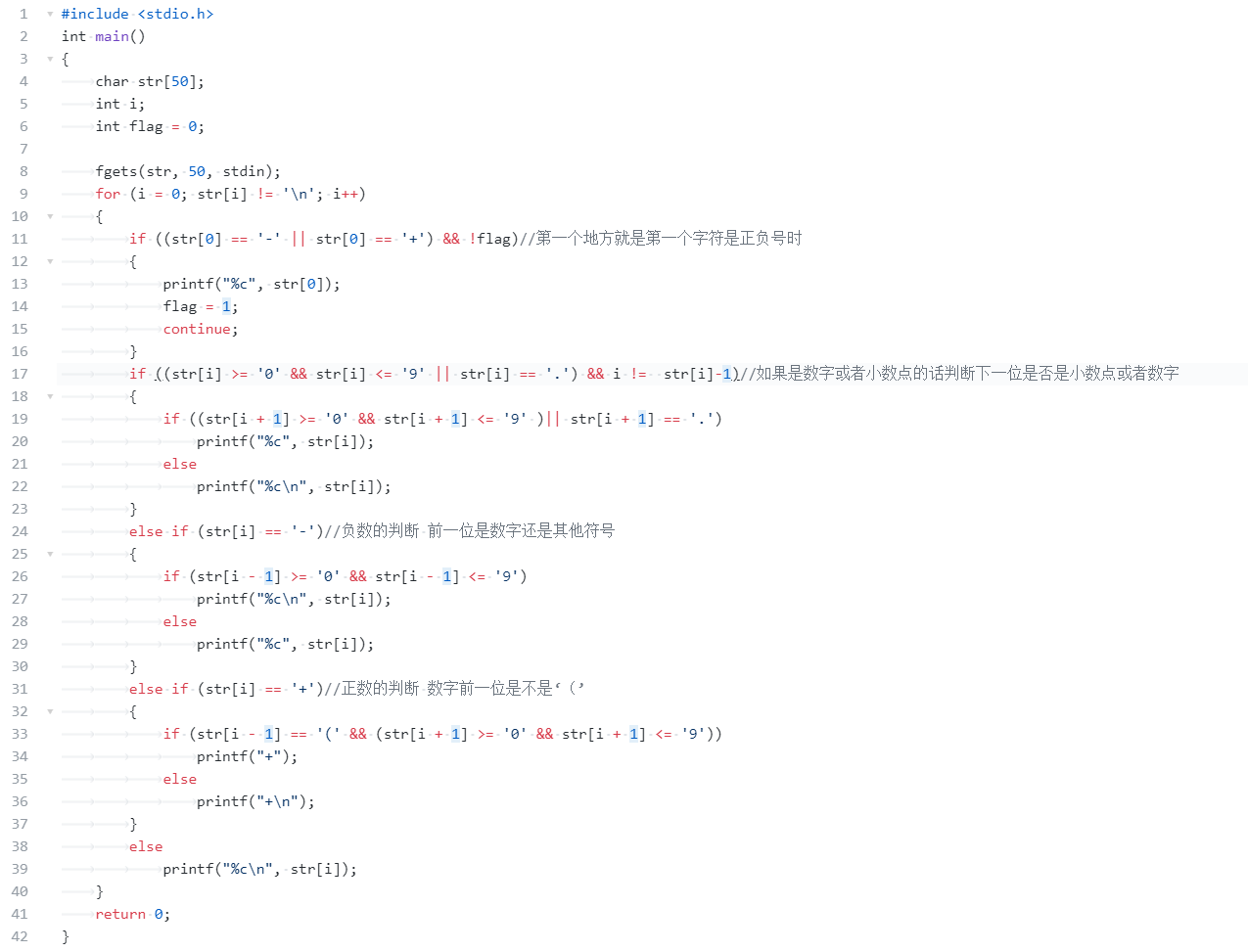2.3.3 请说明和超星视频做法区别，各自优缺点。

posted @ 2020-12-13 22:28  Li-xinguo★  阅读(177)  评论(0编辑  收藏  举报